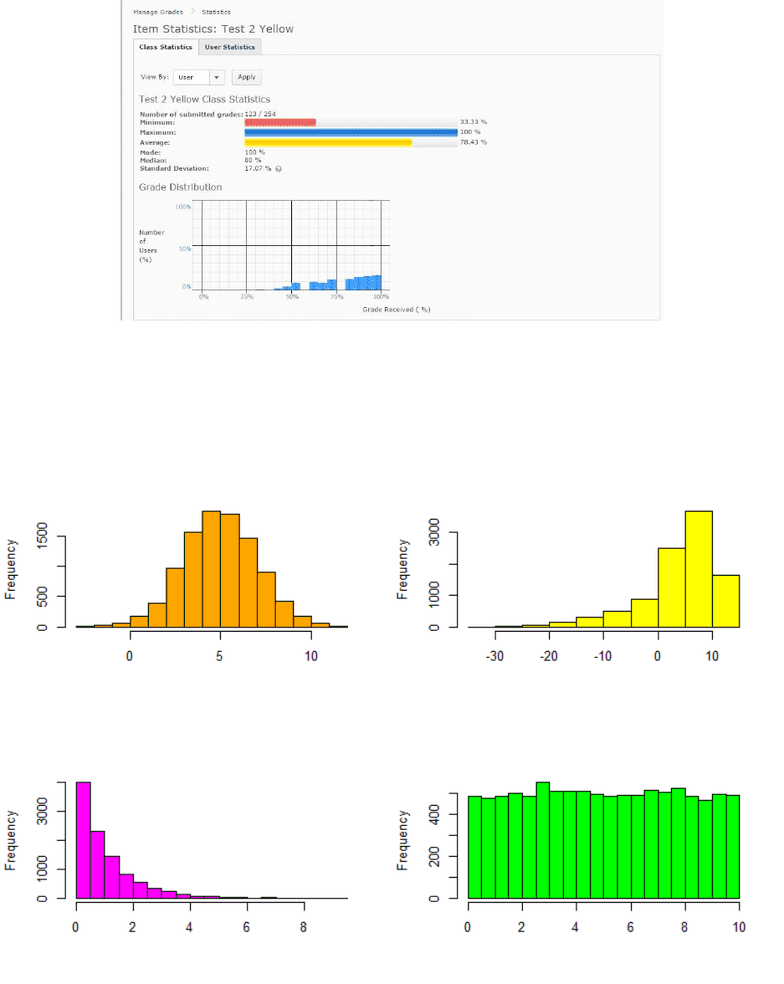# STAT 2060 Lecture Notes - Lecture 2: Box Plot, Bias Of An Estimator, Unimodality

254 views14 pages
School
Department
Course
Professor
STAT*2060: Statistics for Business Decisions
Week 2 Lectures
1 Measures of Central Tendency
The most common measures of central tendency are the mean, median, and mode.
Mean:
The mean is the average of all observations.
When we are working with a sample, the sample mean is denoted ¯x, where:
EXAMPLE: Suppose we have the numbers -2, 4, 8, 13, 19. We can calculate the sample mean as:
¯xis a sample mean, but it is often used to estimate the population mean, µ(“mew”).
The mean uses all data points in its calculations (which is good!), but is therefore sensitive to extreme
values (which is bad!)
EXAMPLE: Suppose we have the numbers -2, 4, 8, 13, 190. The sample mean is:
Median:
The median is the middle value when the data is ordered smallest to largest.
If nodd, median is the middle value. If nis even, median is the average of the two middle values.
EXAMPLE: Suppose we have the numbers 2, -5, 18, 9, 0. We can determine the median as:
1
Average of all data samples (a+b+c/3)
x/={xi/n (i=1)
x={xi/n
=(-2)+4+8+13+19/5
=42/5
=8.4
Check out calculator
x bar used to estimate mew
x bar is statistic
parameter
(-2)+4+8+13+190/5
=213/5
=42.6
190 pulled mean toward it from 8.4-->42.6
Thus extreme values in data set can result in a mean that is not reflective of most of the data.
Unlock document

This preview shows pages 1-3 of the document.
Unlock all 14 pages and 3 million more documents.

Already have an account? Log in
EXAMPLE: Now suppose we have the numbers 2, -5, 18, 9, 0, 11. We can now determine the median as:
The median only uses the values of the middle number(s) in its calculations (which is o.k.), but is
therefore less sensitive to extreme values (which is good!).
EXAMPLE: Consider the values from above, which were 2, -5, 18, 9, 0. The median was found to be
2, and the mean we can calculate to be 4.8. Suppose the value of 9 is changed to 90. The new mean
and median are now
Mode:
The mode is the most frequently occurring observation. It is possible to have one (unimodal), two
(bimodal), or even more (multimodal) modes in a single set of data.
EXAMPLE: Suppose we have the numbers 3, 4, 5, 5, 6, 6, 6, 6, 7, 7, 8, 8, 9. We can determine the
mode as:
The mode is not necessarily that useful, and can be misleading!
2
-5,0,2,9,11,18 therefore (2+9=11/2=5.5) 5.5 is median
-5,0,2,18,90
Median: 2
Mean:105/5=21
Therefore, median not influenced, whereas the mean changes significantly
the data occurring most often, in this case it is 6
Bimodal: 3,4,5,5,5,5,6,6,6,6,7,8 Therefore, both 5 & 6 occur as frequently thus, this is a bimodal sample
Unlock document

This preview shows pages 1-3 of the document.
Unlock all 14 pages and 3 million more documents.

Already have an account? Log inWe can make general conclusions about the location of the mean and median (and even the mode) by
examining the shape of the distribution of the data:
3
Mode doesn't imply Majority, just that number appears most frequently in
sample
Symmetric: mean & median are roughly same
Median: roughly 5
Mean: roughly 5
Left-skewed (Assymmetric): mean less than median
Median: roughly 4.8
Mean: -5
Right-Skewed (Assymmetric)
Median: less than mean
Mean: greater than median
Uniform (Symmetric):
Mean & Median roughly equal
Unlock document

This preview shows pages 1-3 of the document.
Unlock all 14 pages and 3 million more documents.

Already have an account? Log in

# Get access

Grade+
\$10 USD/m
Billed \$120 USD annually
Homework Help
Class Notes
Textbook Notes
40 Verified Answers
Study Guides
1 Booster Class
Class+
\$8 USD/m
Billed \$96 USD annually
Homework Help
Class Notes
Textbook Notes
30 Verified Answers
Study Guides
1 Booster Class